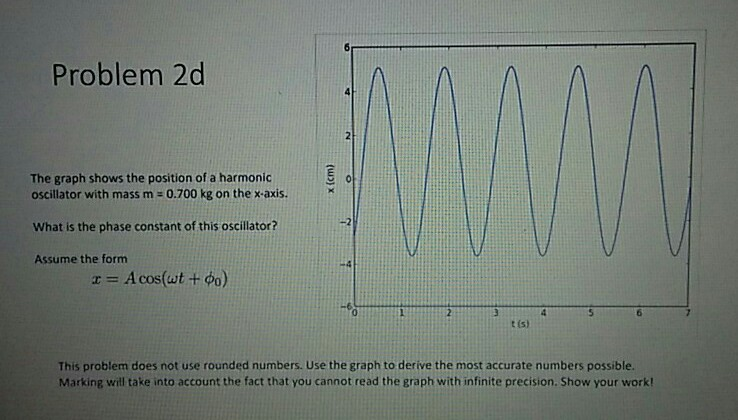# Problem 2d The graph shows the position of a harmonic oscillator with mass m 0.700 kg on the x-axis. What is the phase constant of this oscillator? Assume the form r Acos(wt + 0 t (s) This problem does not use rounded numbers. Use the graph to derive the most accurate numbers possible. Marking will take into account the fact that you cannot read the graph with infinite precision. Show your work!

Questionhelp_outlineImage TranscriptioncloseProblem 2d The graph shows the position of a harmonic oscillator with mass m 0.700 kg on the x-axis. What is the phase constant of this oscillator? Assume the form r Acos(wt + 0 t (s) This problem does not use rounded numbers. Use the graph to derive the most accurate numbers possible. Marking will take into account the fact that you cannot read the graph with infinite precision. Show your work! fullscreen

### Want to see the step-by-step answer?